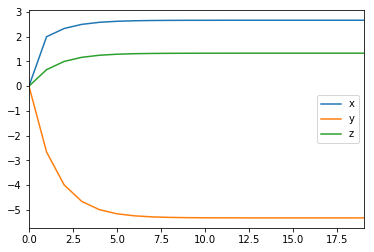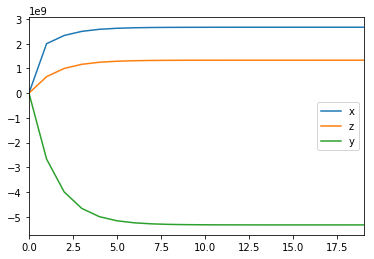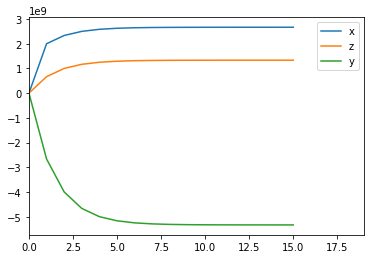# Decentralized Optimization with Blockchains

This tutorial walks through an example of how to implement ADMM on a blockchain, and complements my Devcon3 talk. Github repository is here

# Goal: Use a smart contract to coordinate decentralized optimization¶

This can model federated machine learning or federated optimization problems

We will use the Alternating Direction Method of Multipliers, or ADMM, as the theoretical framework for breaking our problem into ADMM steps. The general idea is:

• Local optmization problems performed locally, based on the last global estimate
• An aggregator gathers together the local solutions, then updates the global variable estimate
• When the global variable converges, we have reached consensus and the problem is solved

We will use Python to perform local computations, then use a smart contract to act as the aggregator step.

### Aggregator:¶

The aggregator must:

• Accept state updates from participants
• Assess whether a sufficient number of participants have submitted updates
• When they have, compute the global variable
• Assess whether the problem is ‘solved’ to sufficient accuracy
• If solved, make the global solution available and alert participants
• If not solved, make the new global estimate availabel and alert participants.

We will first examine a federated/private blockchain, where we have a whitelist of allowable participants and know that we can compute the update when all participants on the whitelist have submitted updates.

# Alternating Direction Method of Multipliers¶

We would like to combine the generous convergence requirements of the method of multipliers (using augmented lagrangians) and the decomposability of dual decomposition.

Assuming we have a problem of the form:
\begin{align} \min_x &\quad f(x) + g(z)\\ \text{s.t.}&\quad Ax+Bz = c \end{align}
which can represent a problem in a single variable which is separable into parts. We form the augmented Lagrangian:
$$L_{\rho}(x,y,z) = f(x)+g(z)+ y^T (Ax + Bz – c) + (\rho/2) ||Ax + Bz – c||^2_2$$
we now consider the update iterations:
\begin{align} x^{k+1} &:= \quad \text{argmin}_x \; L_{\rho}(x ,y^k,z^k) \\ z^{k+1} &:= \quad \text{argmin}_z \; L_{\rho}(x^{k+1},y ,z^k) \\ y^{k+1} &:= \quad y^k + \rho(Ax^{k+1} + Bz^{k+1} – c) \end{align}
Separating the minimization into two steps, each over a single set of variables, is what allows for the decomposition.

### Scaled form¶

A more convenient form for tracking convergence can be attained by considering a residual $r=Ax+Bz-c$ on the constraints, in which case
$$y^T r + \frac{\rho}{2}\lVert r \rVert^2_2 \quad = \quad \frac{\rho}{2}\lVert r + \frac{1}{\rho}y \rVert^2_2 – \frac{1}{2\rho}||y||^2_2 \quad = \quad \frac{\rho}{2}\lVert r + u \rVert^2_2 – \frac{\rho}{2}\lVert u \rVert^2_2$$
where the 2-norm is expanded into a quadratic term to allow for collection of the $y$ terms. This allows the ADMM updates to be expressed as:
\begin{align} x^{k+1} &:= \quad \text{argmin}_x \; f(x) + \frac{\rho}{2}\lVert Ax + Bz^k – c + u^k \rVert^2_2 \\ z^{k+1} &:= \quad \text{argmin}_z \; g(x) + \frac{\rho}{2}\lVert Ax^{k+1} + Bz – c + u^k \rVert^2_2 \\ u^{k+1} &:= \quad u^k + Ax^{k+1} + Bz^{k+1} – c \end{align}

\begin{align} \min_{x,z} & \quad x^2 + 2z^2 \\ \text{s.t.} & \quad x + z = 4 \end{align}

In standard ADMM form, $A=1,\; B=1,\; c=4,\; f=x^2,\; g=2z^2$ and the Augmented Lagrangian is:
\begin{align} L_{\rho}(x,y,z) &= x^2 + 2z^2 + y^T(x+z-c) + (\rho/2)\lVert x+z-c \rVert^2_2\\ &= x^2 + 2z^2 + yx + yz – cy + \frac{\rho}{2} \left( x^2 + z^2 -2cx -2cz + 2xz + c^2 \right) \end{align}
and thus the closed-form solution for the minimizer steps will be:
\begin{align} x^{k+1} &= \frac{1}{2+\rho}\left( \rho c – y^k – \rho z^k \right)\\ z^{k+1} &= \frac{1}{4+\rho}\left( \rho c – y^k – \rho x^{k+1} \right)\\ y^{k+1} &= y^k + \rho x^{k+1} + \rho z^{k+1} – \rho c \end{align}

In :
import pandas as pd
import matplotlib.pyplot as plt
% matplotlib inline
from cvxpy import *

In :
## Use an off-the-shelf constrained optimization solver (CvxPy)
x = Variable()
z = Variable()

obj = Minimize(x**2 + 2*z**2)
constraints = [x + z == 4]
result = Problem(obj,constraints).solve()

print("Solution at x=%.3f, z=%.3f"%(x.value,z.value))

Solution at x=2.667, z=1.333

In :
## Centralized Form

iterations = 20
x,y,z = [[float('NaN')]*iterations for i in range(3)]
x,y,z = [0,0,0]

rho = 2
c = 4

for i in xrange(1,iterations):
x[i] = 1/(2.+rho) * (c*rho - y[i-1] - rho*z[i-1])
z[i] = 1/(4.+rho) * (c*rho - y[i-1] - rho*x[i])
y[i] = y[i-1] + rho * (x[i] + z[i] - c)

print("Solution at x=%.3f, z=%.3f"%(x[-1],z[-1]))
df = pd.DataFrame([x,y,z],index=['x','y','z']).T
df.plot()

Solution at x=2.667, z=1.333

Out:
<matplotlib.axes._subplots.AxesSubplot at 0x1166f0310># Super-Simple Aggregator:¶

This is based on AggSimple.sol

Aggregator runs the simple conventional ADMM update step: $y^+ = y + \rho(Ax+Bz-c)$

No checks on the iteration number submitted from each of the participants, or the stopping number- this could keep getting called forever!

### Infrastructure setup:¶

This requires the following:

My process for deploying:

• Install Python packages including the patched version from github of ethjsonrpc
• Run $jupyter notebook and run this iPython notebook • In a separate terminal window, run $ testrpc to get a testrpc network going
• In a separate terminal window, run $truffle migrate --reset to compile the contracts and deploy them to the test network Caveats: This has not been deployed on the main Ethereum network (or even the testnet); it also has not been run through any security checks. The goal is for transparency and ease of teaching/exposition, not robustness or security! In : from ethjsonrpc import EthJsonRpc, BadResponseError conn = EthJsonRpc('127.0.0.1', 8545)  In : x_addr = conn.eth_accounts() z_addr = conn.eth_accounts() contractAddr = open('AggSimpleAddress.txt','rb').read() contractAddr  Out: '0xa5ab9b0b411ee608d4aecffaeea8d653aa6f61ad' In : iterations = 20; resultDf = pd.DataFrame(index = range(iterations),columns=['x','z','y']) x_addr = conn.eth_accounts() z_addr = conn.eth_accounts() #### ADMM loop: Update x, update z, update y # General information: this can be called separately by each participant rho = conn.call(contractAddr,'rho()',[],['int256']) c = conn.call(contractAddr,'c()',[],['int256']) i = 0 resultDf.loc[0,:] = [0,0,0] for i in range(1, iterations): ### X LOCAL CALCULATION: need to retrieve z and y z = conn.call(contractAddr,'z()',[],['int256']) y = conn.call(contractAddr,'y()',[],['int256']) x = int( 1/(2.+rho) * (c*rho - y - rho*z) ) # Submit the x-update tx = conn.call_with_transaction(x_addr, contractAddr, 'setX(int256)',[x]) while conn.eth_getTransactionReceipt(tx) is None: time.sleep(0.1) # Wait for the transaction to be mined and state changes to take effect # Z LOCAL CALCULATION: This needs to processed after the x-update x = conn.call(contractAddr,'x()',[],['int256']) y = conn.call(contractAddr,'y()',[],['int256']) z = int( 1/(4.+rho) * (c*rho - y - rho*x) ) tx = conn.call_with_transaction(z_addr, contractAddr, 'setZ(int256)',[z]) while conn.eth_getTransactionReceipt(tx) is None: time.sleep(0.2) # Wait for the transaction to be mined and state changes to take effect # Update y: this happens on the blockchain tx = conn.call_with_transaction(x_addr, contractAddr, 'updateY()',[]) while conn.eth_getTransactionReceipt(tx) is None: time.sleep(0.1) # Wait for the transaction to be mined and state changes to take effect # Save the values of the state for this iteration: y = conn.call(contractAddr,'y()',[],['int256']) resultDf.loc[i,:] = [x,z,y] resultDf.plot()  Out: <matplotlib.axes._subplots.AxesSubplot at 0x1187919d0>In [ ]: # Use this if you want to reset the variable values to run it again tx = conn.call_with_transaction(z_addr, contractAddr, 'setX(int256)',) tx = conn.call_with_transaction(z_addr, contractAddr, 'setZ(int256)',) tx = conn.call_with_transaction(z_addr, contractAddr, 'setY(int256)',)  # Manage access and make variable updates automatic¶ The contract Aggregator.sol extends the above approach in two important ways: • Making sure that each participant can only update the variables which they are responsible for • Checks whether the solution has converged to within acceptable bounds and we can consider the problem ‘solved’ There are two stopping criteria which we want to satisfy:$||r^k||_2 \leq \epsilon^{\text{pri}},\; ||s^k||_2 \leq \epsilon^{\text{dual}}$• Primal Residual:$\quad r^{k+1} = Ax^{k+1} + Bz^{k+1} – c$• Dual Residual:$\quad s^{k+1} = \rho A^T B(z^{k+1} – z^k\$)

These tolerances can be set in the smart contract by adjusting the variables eps_pri and eps_dual

In :
x_addr = conn.eth_accounts()


Out:
'0xe192a96f1ebb53aa25fdd4f7879bf78b6649e48f'
In :
iterations = 20;
resultDf = pd.DataFrame(index = range(iterations),columns=['x','z','y'])

updateXfcn = 'submitValue(int256,uint16)'
updateZfcn = 'submitValue(int256,uint16)'
updateYfcn = None

#### ADMM loop: Update x, update z, update y
# General information: this can be called separately by each participant

i = 0
resultDf.loc[0,:] = [0,0,0]

for i in range(1, 20):
### X LOCAL CALCULATION: need to retrieve z and y
x = int( 1/(2.+rho) * (c*rho - y - rho*z) )
# Submit the x-update
while conn.eth_getTransactionReceipt(tx) is None:
time.sleep(0.1) # Wait for the transaction to be mined and state changes to take effect

# Z LOCAL CALCULATION: This needs to processed after the x-update
z = int( 1/(4.+rho) * (c*rho - y - rho*x) )
while conn.eth_getTransactionReceipt(tx) is None:
time.sleep(0.2) # Wait for the transaction to be mined and state changes to take effect

# Update y: this happens on the blockchain
if updateYfcn is not None:
while conn.eth_getTransactionReceipt(tx) is None:
time.sleep(0.1) # Wait for the transaction to be mined and state changes to take effect

# Save the values of the state for this iteration:
resultDf.loc[i,:] = [x,z,y]
print("Primal residual r=%s,\t Dual residual s=%s, \t problemSolved is %s"%(r,s,solved))
if solved: break

resultDf.plot()

Primal residual r=1333333334,	 Dual residual s=1333333332, 	 problemSolved is False
Primal residual r=666666666,	 Dual residual s=666666668, 	 problemSolved is False
Primal residual r=333333334,	 Dual residual s=333333332, 	 problemSolved is False
Primal residual r=166666666,	 Dual residual s=166666668, 	 problemSolved is False
Primal residual r=83333334,	 Dual residual s=83333332, 	 problemSolved is False
Primal residual r=41666666,	 Dual residual s=41666668, 	 problemSolved is False
Primal residual r=20833334,	 Dual residual s=20833332, 	 problemSolved is False
Primal residual r=10416666,	 Dual residual s=10416668, 	 problemSolved is False
Primal residual r=5208334,	 Dual residual s=5208332, 	 problemSolved is False
Primal residual r=2604166,	 Dual residual s=2604168, 	 problemSolved is False
Primal residual r=1302084,	 Dual residual s=1302082, 	 problemSolved is False
Primal residual r=651042,	 Dual residual s=651042, 	 problemSolved is False
Primal residual r=325520,	 Dual residual s=325522, 	 problemSolved is False
Primal residual r=162761,	 Dual residual s=162760, 	 problemSolved is False
Primal residual r=81380,	 Dual residual s=81380, 	 problemSolved is True

Out:
<matplotlib.axes._subplots.AxesSubplot at 0x11878f790>### One comment

1.Alvaro says:

I would like to thank you for this excellent tutorial and for the accompanying Github code! It has been most useful for following your Optimal Power Flow paper and adapting it for my own research 🙂